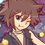# Algebra (Thailand Math POSN 3rd round)

Write a full solution.

1. Let $P(x),Q(x)$ be real polynomials (real coefficients) with leading coefficient $1$ or $-1$ such that $deg(P(x)) > deg(Q(x))$, find the number of solutions $(P(x),Q(x))$ to $P(x)^{2}+Q(x)^{2} = x^{8}+1$. If possible, find each forms of solution.

2. Let $f(x) = \displaystyle \left(\frac{x^{5}}{5} + \frac{x^{4}}{2} + \frac{x^{3}}{3} - \frac{1}{30}\right) - \left\lfloor \frac{x^{5}}{5} + \frac{x^{4}}{2} + \frac{x^{3}}{3} - \frac{1}{30}\right\rfloor$. Find all possible values of $f(n)$ where $n$ is a positive integer. (Where $\lfloor x \rfloor$ is a floor function, and defined to be $\lfloor x \rfloor \leq x < \lfloor x \rfloor +1$)

3. Find all real polynomials $P(x)$ that satisfy $P(a-b)+P(b-c)+P(c-a) = 2P(a+b+c)$ for all reals $a,b,c$ that satisfy $ab+bc+ca = 0$.

This note is part of Thailand Math POSN 3rd round 2015Note by Samuraiwarm Tsunayoshi
6 years, 3 months ago

This discussion board is a place to discuss our Daily Challenges and the math and science related to those challenges. Explanations are more than just a solution — they should explain the steps and thinking strategies that you used to obtain the solution. Comments should further the discussion of math and science.

When posting on Brilliant:

• Use the emojis to react to an explanation, whether you're congratulating a job well done , or just really confused .
• Ask specific questions about the challenge or the steps in somebody's explanation. Well-posed questions can add a lot to the discussion, but posting "I don't understand!" doesn't help anyone.
• Try to contribute something new to the discussion, whether it is an extension, generalization or other idea related to the challenge.

MarkdownAppears as
*italics* or _italics_ italics
**bold** or __bold__ bold
- bulleted- list
• bulleted
• list
1. numbered2. list
1. numbered
2. list
Note: you must add a full line of space before and after lists for them to show up correctly
paragraph 1paragraph 2

paragraph 1

paragraph 2

[example link](https://brilliant.org)example link
> This is a quote
This is a quote
    # I indented these lines
# 4 spaces, and now they show
# up as a code block.

print "hello world"
# I indented these lines
# 4 spaces, and now they show
# up as a code block.

print "hello world"
MathAppears as
Remember to wrap math in $$ ... $$ or $ ... $ to ensure proper formatting.
2 \times 3 $2 \times 3$
2^{34} $2^{34}$
a_{i-1} $a_{i-1}$
\frac{2}{3} $\frac{2}{3}$
\sqrt{2} $\sqrt{2}$
\sum_{i=1}^3 $\sum_{i=1}^3$
\sin \theta $\sin \theta$
\boxed{123} $\boxed{123}$

Sort by:

ooooh, I like question 3 :) I tend to have a soft spot for such functional equations.

Staff - 6 years, 3 months ago

Sir try This and this $\ddot\smile$

- 6 years, 3 months ago